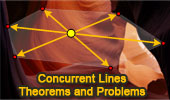# Geometry, Concurrent Lines, Theorems and Problems

## Concurrent Lines - Table of Content - Page 1Clawson Point.
Orthic and Extangents Triangles, Concurrent lines.

Kosnita's Theorem.
Circumcenters, Concurrent lines.

Isogonic-Jacobi Theorem
Using TracenPoche Dynamic Geometry Software

Dynamic Geometry 1478.
Cyclocevian, Reuschle-Terquem Theorem, Concurrent Cevians, Triangle, Circumcircle, Secant line, Step-by-step Illustration. GeoGebra, iPad.

Dynamic Geometry 1473.
Kosnita's Theorem, Triangle, Four Circumcenters, Concurrent Line, Step-by-step Illustration.

Dynamic Geometry 1471.
Equilateral Triangle, Inside/Outside Point, Incenters, Tangency Points, Concurrent Lines, Step-by-step Illustration.

Dynamic Geometry 1451.
Orthopole of a Line. Step-by-step illustration using GeoGebra.

Geometry Problem 1425.
Two Squares, Collinear Points, Concurrent Lines.

Geometry Problem 1400.
Triangle with three rectangles on the sides, Perpendicular Bisectors, Concurrency.

Geometry Problem 1396.
Triangle with three rectangles on the sides, Vertices, Perpendicular lines, Concurrency.

Geometry Problem 1395.
Triangle with three rectangles on the sides, Midpoints, Perpendicular lines, Concurrency.

Geometry Problem 1394.
Three Circles and Three Pair of Common Internal Tangents, Concurrency.

Geometry Problem 1301.
Arbelos, Semicircles, Diameters, Circle, Incircle, Incenter, Square, Midpoint, Concurrency.

Geometry Problem 1300.
Arbelos, Semicircles, Diameters, Circle, Incircle, Tangent, Perpendicular, Concurrent Lines.

Geometry Problem 1293.
Triangle, Altitudes, Two Squares, Center, Concurrent Lines, Midpoint.

Geometry Problem 1289.
Triangle, Cevian, Concurrency, Measurement, Metric Relations.

Geometry Problem 1264.
Triangle, Exterior Angle Bisector, Circumcircle, Circle, Perpendicular, 90 Degrees, Concurrent Lines.

Geometry Problem 1260.
Triangle, Internal Angle Bisector, Circumcircle, Circle, Perpendicular, 90 Degrees, Concurrent Lines.

Geometry Problem 1253.
Triangle, Orthocenter, Circle, Circumcircle, Altitude, Perpendicular, 90 Degree, Concurrency.

Geometry Problem 1242.
Quadrilateral, Four Interior and Exterior Angle Bisectors, Collinearity, Concurrent Lines. Mobile Apps.

Geometry Problem 1237
IMO 2016, Problem 1, Triangle, Congruence, Parallel Lines, Midpoint, Concurrency.

Geometry Problem 1235
Triangle, Cevians, Concurrency, Circle, Circumcircle.

Geometry Problem 1212
Equilateral Triangle, Equilateral Hexagon, Concurrent Lines.

Geometry Problem 1184
Right Triangle, Square, Inscribed Circle, Tangent, Concurrent Lines.

Geometry Problem 1176
Cyclic Quadrilateral, Diagonals, Six Diameters, Circles, Collinear Points, Concurrent Lines.

Geometry Problem 1141.
Inscribed Pentagon, Circle, Triangle, Congruence, Midpoint, Concurrency.

Geometry Problem 1139.
Triangle, Circumcircle, Angle Bisector, Parallel Lines, 90 Degrees, Concurrent Lines.

Geometry Problem 1088.
Triangles, Perpendicular, Concurrent Lines.

Geometry Problem 1047.
Parallelogram, Parallel Lines, Concurrent Lines, Diagonals.

Geometry Problem 1012.
Equilateral Triangle, Incenters, Inscribed Circles, Perpendicular, Concurrent Lines.

Geometry Problem 1007.
Hexagon, Midpoint, Triangle, Median, Centroid, Concurrency.

Geometry Problem 1004.
Triangle with Squares, Perpendicular Bisectors, Concurrent Lines.

Geometry Problem 994.
Trapezoid, Midpoints of the bases, Concurrent Lines.

Geometry Problem 981.
Triangle, Concurrent Cevians, Midpoints, Area, Hexagon.

Geometry Problem 979.
Triangle, Cevian, Angle Bisector, Concurrency, 90 Degrees, Perpendicular.

Geometry Problem 974.
'Begonia Theorem', Cevian Triangle, Reflection of a point in a line, Concurrency of Lines. GeoGebra, HTML5 Animation for Tablets.

Geometry Problem 945.
Triangle, Concurrent Lines, Cevians, Perpendicular, Angle Bisector.

Geometry Problem 929.
Triangle, Orthocenter, Circumcircle, Reflection, Concurrency.

Geometry Problem 928.
Triangle, Midpoint, Median, Cevian, Concurrency, Area.

Excenter. Index.
Concurrent angle bisectors

Circumcenter. Index.
Concurrent perpendicular bisectors.

Orthocenter Index.
Concurrent altitudes.

Centroid /Barycenter. Index.
Concurrent medians.

Triangle, Medians, Six Circumcenters Concyclic. Dynamic Geometry.
Step-by-Step construction, Manipulation, and animation.
Concurrent medians..

Trapezoid, Triangle, Diagonals, Midpoints. Dynamic Geometry.
Step-by-Step construction, Manipulation, and animation.
Concurrent lines.

Triangle and Squares Theorem 1: with Interactive Geometry Software
Step-by-Step construction, Manipulation, and animation.
Concurrent lines.

Gergonne's Theorem: Triangle and Concurrent Cevians.

Taylor Circle Theorem Using TracenPoche Dynamic Software
Step-by-Step construction, Manipulation, and animation.
Henry Martyn Taylor and the blind student of mathematics
Concurrent altitudes.

Interactive Orthopole of a Triangle: Using TracenPoche Dynamic Geometry Software, Online Step-by-Step construction, manipulation, and animation.
Concurrent perpendiculars.

Schiffler Point: Concurrent Four Euler Lines with interactive animation and manipulation.

Incenter, Excenter, Incircle, Excircle Using TracenPoche Dynamic Software
Step-by-Step construction, Manipulation, and animation.
Concurrent angle bisectors.

Three Tangent Circles Theorem Using TracenPoche Dynamic Software
Step-by-Step construction, Manipulation, and animation.
Concurrent common tangent lines

Three Circles Theorem Using TracenPoche Dynamic Software.
Concurrent common chords

Symmedian (Lemoine) Point
Triangle Center

Nagel Point Theorem. Proof.
Concurren lines.

Gergonne Point Theorem. Concurrency. Interactive proof with animation.
Key concept: Ceva's Theorem.

The Bevan Point The circumcenter of the excentral triangle. Illustration with animation.
Concurrent perpendiculars.

Ceva's Theorem. Concurrency. Interactive proof with animation. Key concept: Menelaus Theorem.

Go to page: Previous | 1 | 2 | 3 | 4 | 5 | Next